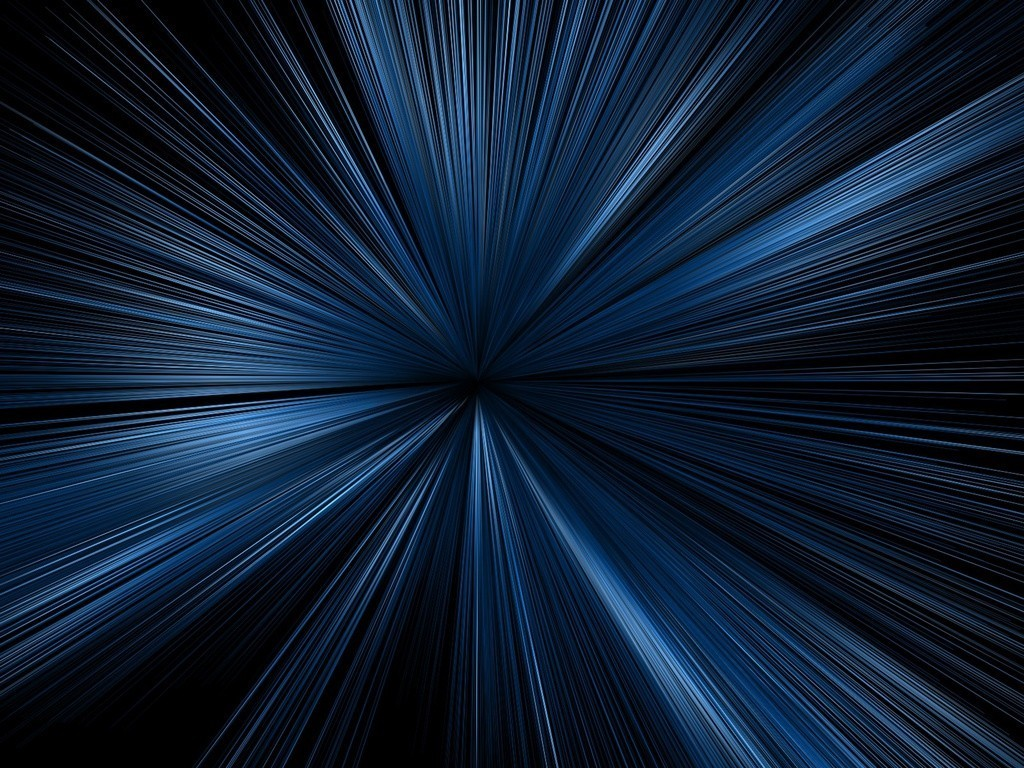# Light Meter

In Physics by Brian Koberlein0 Comments

It is impossible to measure the speed of light, and it has been impossible since 1983.  No, the behavior of light didn’t suddenly change in 1983.  What changed was the way we define the length of a meter.

Measuring the speed of light has always been tricky because of its tremendous speed.  It wasn’t until the 1600s that astronomers such as Romer and Huygens measured its speed by observing the moons of Jupiter.   Their approach was to carefully measure the transits of Jupiter’s moon Io very carefully when the Earth was moving toward Jupiter and when it was moving away from Jupiter.  By measuring the difference in those times they were able to calculate a speed of around 200,000 kilometers per second.  Their value is about a third lower than the actual value, but it’s astoundingly accurate given the crudeness of their equipment.

By the 1900s, we were able to use the interference of light waves to get very precise measurements of light speed.  When lasers were introduced, these interferometry measurements became more precise than the definition of a standard meter.  This meant the uncertainty of the speed of light lay not in the measurement, but in the uncertainty of how long a meter was.  So in 1983, it was decided to define the meter from the speed of light.  This made the speed of light (in a vacuum) 299,792,458 m/s by definition.  As a result, if you try to measure the speed of light, you are actually measuring the length of a meter.

Of course this raises an interesting question.  If we’ve defined the speed of light in a vacuum to be a constant, doesn’t that mean that effects such as the expansion of the universe are just the same as the slowing of the speed of light?  The answer is actually no.  We can still measure changes in light regardless of how we define our units.  For example, the famous Michelson-Morley experiment (which demonstrated that the speed of light constant) was first performed in the late 1800s, before a precise speed of light was even known.

So we don’t lose anything by our new definition.  What we gain is a better way to measure distance, which is central to building better astronomical equipment.